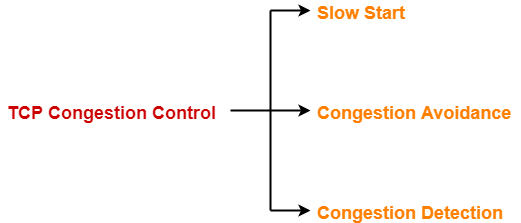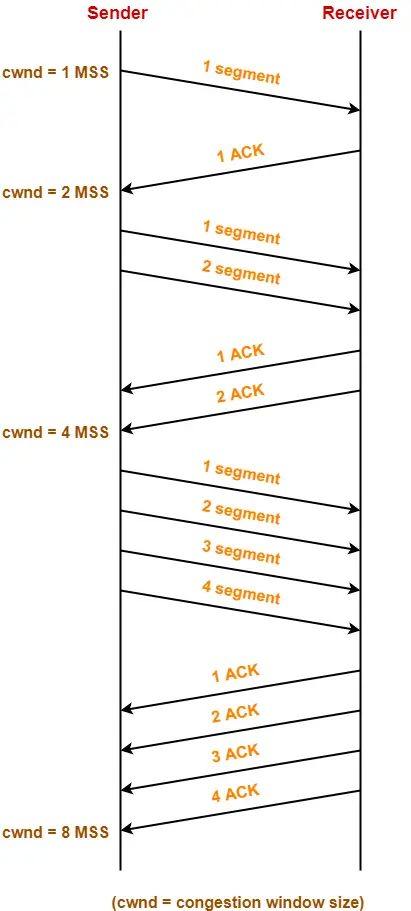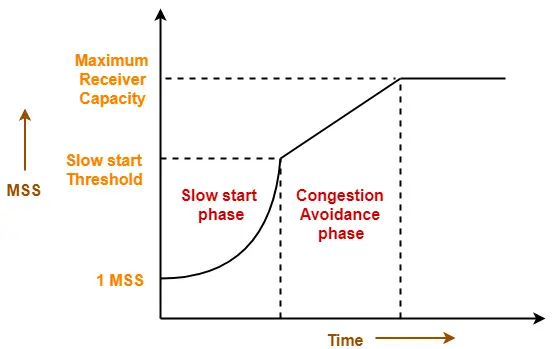## TCP Congestion Control-

Before you go through this article, make sure that you have gone through the previous article on TCP Congestion Control.

TCP congestion control policy consists of following three phases-1. Slow Start
2. Congestion Avoidance
3. Congestion Detection

In this article, we will discuss practice problems on TCP Congestion Control.

## Problem-01:

The growth of congestion window takes place-

1. Infinitely
2. Up to Threshold
3. Up to the size of receiver’s window
4. Up to timeout

## Solution-

Option (C) is correct.

## Problem-02:

Consider the effect of using slow start on a line with a 10 msec RTT and no congestion. The receiver window is 24 KB and the maximum segment size is 2 KB. How long does it take before the first full window can be sent?

## Solution-

Given-

• Receiver window size = 24 KB
• Maximum Segment Size = 2 KB
• RTT = 10 msec

Receiver window size in terms of MSS

= Receiver window size / Size of 1 MSS

= 24 KB / 2 KB

= 12 MSS

### Slow Start Threshold-

Slow start Threshold

= Receiver window size / 2

= 12 MSS / 2

= 6 MSS

### Slow Start Phase-

• Window size at the start of 1st transmission = 1 MSS
• Window size at the start of 2nd transmission = 2 MSS
• Window size at the start of 3rd transmission = 4 MSS
• Window size at the start of 4th transmission = 6 MSS

Since the threshold is reached, so it marks the end of slow start phase.

Now, congestion avoidance phase begins.

### Congestion Avoidance Phase-

• Window size at the start of 5th transmission = 7 MSS
• Window size at the start of 6th transmission = 8 MSS
• Window size at the start of 7th transmission = 9 MSS
• Window size at the start of 8th transmission = 10 MSS
• Window size at the start of 9th transmission = 11 MSS
• Window size at the start of 10th transmission = 12 MSS

From here,

• Window size at the end of 9th transmission or at the start of 10th transmission is 12 MSS.
• Thus, 9 RTT’s will be taken before the first full window can be sent.

So,

Time taken before the first full window is sent

= 9 RTT’s

= 9 x 10 msec

= 90 msec

## Problem-03:

Consider an instance of TCP’s Additive Increase Multiplicative Decrease (AIMD) algorithm where the window size at the start of slow start phase is 2 MSS and the threshold at the start of first transmission is 8 MSS. Assume that a time out occurs during the fifth transmission. Find the congestion window size at the end of tenth transmission.

1. 8 MSS
2. 14 MSS
3. 7 MSS
4. 12 MSS

## Solution-

Given-

• Window size at the start of slow start phase = 2 MSS
• Threshold at the start of first transmission = 8 MSS
• Time out occurs during 5th transmission

### Slow Start Phase-

• Window size at the start of 1st transmission = 2 MSS
• Window size at the start of 2nd transmission = 4 MSS
• Window size at the start of 3rd transmission = 8 MSS

Since the threshold is reached, so it marks the end of slow start phase.

Now, congestion avoidance phase begins.

### Congestion Avoidance Phase-

• Window size at the start of 4th transmission = 9 MSS
• Window size at the start of 5th transmission = 10 MSS

It is given that time out occurs during 5th transmission.

TCP reacts by-

• Setting the slow start threshold to half of the current congestion window size.
• Decreasing the congestion window size to 2 MSS (Given value is used).
• Resuming the slow start phase.

So now,

• Slow start threshold = 10 MSS / 2 = 5 MSS
• Congestion window size = 2 MSS

### Slow Start Phase-

• Window size at the start of 6th transmission = 2 MSS
• Window size at the start of 7th transmission = 4 MSS
• Window size at the start of 8th transmission = 5 MSS

Since the threshold is reached, so it marks the end of slow start phase.

Now, congestion avoidance phase begins.

### Congestion Avoidance Phase-

• Window size at the start of 9th transmission = 6 MSS
• Window size at the start of 10th transmission = 7 MSS
• Window size at the start of 11th transmission = 8 MSS

From here,

Window size at the end of 10th transmission

= Window size at the start of 11th transmission

= 8 MSS

Thus, Option (A) is correct.

## Problem-04:

Suppose that the TCP congestion window is set to 18 KB and a time out occurs. How big will the window be if the next four transmission bursts are all successful? Assume that the MSS is 1 KB.

## Solution-

### Congestion Window Size-

Congestion window size in terms of MSS

= 18 KB / Size of 1 MSS

= 18 KB / 1 KB

= 18 MSS

### Reaction Of TCP On Time Out-

TCP reacts by-

• Setting the slow start threshold to half of the current congestion window size.
• Decreasing the congestion window size to 1 MSS.
• Resuming the slow start phase.

So now,

• Slow start threshold = 18 MSS / 2 = 9 MSS
• Congestion window size = 1 MSS

### Slow Start Phase-

• Window size at the start of 1st transmission = 1 MSS
• Window size at the start of 2nd transmission = 2 MSS
• Window size at the start of 3rd transmission = 4 MSS
• Window size at the start of 4th transmission = 8 MSS
• Window size at the start of 5th transmission = 9 MSS

Thus, after 4 successful transmissions, window size will be 9 MSS or 9 KB.

## Problem-05:

On a TCP connection, current congestion window size is 4 KB. The window advertised by the receiver is 6 KB. The last byte sent by the sender is 10240 and the last byte acknowledged by the receiver is 8192.

### Part-01:

The current window size at the sender is ____.

1. 2048 B
2. 4096 B
3. 6144 B
4. 8192 B

### Part-02:

The amount of free space in the sender window is ____.

1. 2048 B
2. 4096 B
3. 6144 B
4. 8192 B

## Solution-

### Part-01:

Sender window size

= min (Congestion window size, Receiver window size)

= min(4KB , 6KB)

= 4 KB

= 4096 B

Thus, Option (B) is correct.

### Part-02:

Given-

• Last byte acknowledged by the receiver = 8192
• Last byte sent by the sender = 10240

From here,

• It means bytes from 8193 to 10240 are still present in the sender’s window.
• These bytes are waiting for their acknowledgement.
• Total bytes present in sender’s window = 10240 – 8193 + 1 = 2048 bytes.

From here,

Amount of free space in sender’s window currently

= 4096 bytes – 2048 bytes

= 2048 bytes

This indicates that half of the sender’s window is currently empty.

Thus, Option (A) is correct.

Next Article- TCP Timers

Get more notes and other study material of Computer Networks.

Watch video lectures by visiting our YouTube channel LearnVidFun.

## Congestion in Network-

 Congestion refers to a network state where-The message traffic becomes so heavy that it slows down the network response time.

• Congestion is an important issue that can arise in Packet Switched Network.
• Congestion leads to the loss of packets in transit.
• So, it is necessary to control the congestion in network.
• It is not possible to completely avoid the congestion.

## Congestion Control-

Congestion control refers to techniques and mechanisms that can-

• Either prevent congestion before it happens
• Or remove congestion after it has happened

Now, let us discuss how congestion is handled at TCP.

## TCP Congestion Control-

 TCP reacts to congestion by reducing the sender window size.

The size of the sender window is determined by the following two factors-

2. Congestion window size

• Sender should not send data greater than receiver window size.
• Otherwise, it leads to dropping the TCP segments which causes TCP Retransmission.
• So, sender should always send data less than or equal to receiver window size.
• Receiver dictates its window size to the sender through TCP Header.

## 2. Congestion Window-

• Sender should not send data greater than congestion window size.
• Otherwise, it leads to dropping the TCP segments which causes TCP Retransmission.
• So, sender should always send data less than or equal to congestion window size.
• Different variants of TCP use different approaches to calculate the size of congestion window.
• Congestion window is known only to the sender and is not sent over the links.

So, always-

 Sender window size = Minimum (Receiver window size, Congestion window size)

## TCP Congestion Policy-

TCP’s general policy for handling congestion consists of following three phases-1. Slow Start
2. Congestion Avoidance
3. Congestion Detection

## 1. Slow Start Phase-

• Initially, sender sets congestion window size = Maximum Segment Size (1 MSS).
• After receiving each acknowledgment, sender increases the congestion window size by 1 MSS.
• In this phase, the size of congestion window increases exponentially.

The followed formula is-

 Congestion window size = Congestion window size + Maximum segment size

This is shown below-• After 1 round trip time, congestion window size = (2)1 = 2 MSS
• After 2 round trip time, congestion window size = (2)2 = 4 MSS
• After 3 round trip time, congestion window size = (2)3 = 8 MSS and so on.

 This phase continues until the congestion window size reaches the slow start threshold. Threshold= Maximum number of TCP segments that receiver window can accommodate / 2= (Receiver window size / Maximum Segment Size) / 2

## 2. Congestion Avoidance Phase-

After reaching the threshold,

• Sender increases the congestion window size linearly to avoid the congestion.
• On receiving each acknowledgement, sender increments the congestion window size by 1.

The followed formula is-

 Congestion window size = Congestion window size + 1

This phase continues until the congestion window size becomes equal to the receiver window size.## 3. Congestion Detection Phase-

When sender detects the loss of segments, it reacts in different ways depending on how the loss is detected-

### Case-01: Detection On Time Out-

• Time Out Timer expires before receiving the acknowledgement for a segment.
• This case suggests the stronger possibility of congestion in the network.
• There are chances that a segment has been dropped in the network.

### Reaction-

In this case, sender reacts by-

• Setting the slow start threshold to half of the current congestion window size.
• Decreasing the congestion window size to 1 MSS.
• Resuming the slow start phase.

### Case-02: Detection On Receiving 3 Duplicate Acknowledgements-

• Sender receives 3 duplicate acknowledgements for a segment.
• This case suggests the weaker possibility of congestion in the network.
• There are chances that a segment has been dropped but few segments sent later may have reached.

### Reaction-

In this case, sender reacts by-

• Setting the slow start threshold to half of the current congestion window size.
• Decreasing the congestion window size to slow start threshold.
• Resuming the congestion avoidance phase.

Next Article- Practice Problems On TCP Congestion Control

Get more notes and other study material of Computer Networks.

Watch video lectures by visiting our YouTube channel LearnVidFun.